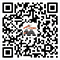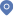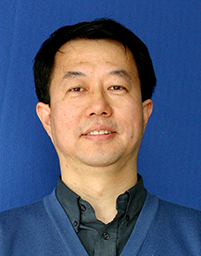ProfessorHome - PeopleAihui Zhou,  Professor

• Research direction：

Finite element method, eigenvalue problem, chaotic dynamics
• Representative work：

``` X. Dai, Q. Wang, and A. Zhou, Gradient flow based discretized Kohn-Sham density functional theory, Multiscale Model. Simul., 18(2020).

 H. Chen, X. Gong, L. He, Z. Yang, and A. Zhou ,  Numerical analysis of finite dimensional approximations of Kohn-Sham models, Adv. Comput. Math., 38 (2013).

 A. Zhou, Hohenberg-Kohn theorem for Coulomb type systems and its generalization, J. Math. Chem., 50(2012).

 A. Zhou, An analysis for finite dimensional approximations for the ground state solution of Bose-Einstein condensates, Nonlinearity, 17(2004).

 J. Xu and A. Zhou, Local and parallel finite element algorithms based on two-grid discretizations, Math. Comput., 69(2000).

```
• Contact information：

Office：Room 715, South Building Telephone：0086-10-82541934 Email：azhou@lsec.cc.ac.cn